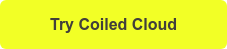# Parquet to CSV: Convert Many Parquet Files to a Single CSV using Python

January 10, 2022

This post demonstrates how to read multiple Parquet files and write them into a single CSV file with just two lines of code. Let’s start by diving right into the parquet to csv syntax and then build a reproducible example you can run on your machine.

Suppose you have the following Parquet files stored on disk:

my_folder/

File1.parquet

File2.parquet

File3.parquet

Here’s how you can convert Parquet to CSV:

```import dask.dataframe as dd

ddf.to_csv("df_all.csv",
single_file=True,
index=False
)```

Let’s turn our attention to creating some Parquet files so you can experiment with this script on your local machine.

Note that if you’re working with medium to large datasets, you might not want to write to a single CSV because it inhibits parallel read/write operations. In that case, scroll down to the end of the post where you’ll learn how to write to multiple CSV files using Dask.

## Create Multiple Parquet Files

We’ll start by creating some DataFrames and writing them to 3 separate Parquet files using a simple `for` loop. Each DataFrame will contain 3 rows and 4 columns and will be filled with random integers between 0 and 100.

```import pandas as pd
import numpy as np

# use the recommended method for generating random integers with NumPy
rng = np.random.default_rng()

# generate 3 dataframes with similar filenames
for i in range(3):
df = pd.DataFrame(rng.integers(0, 100, size=(3, 4)), columns=list('ABCD'))
df.to_parquet(f"df_{i}.parquet")```

If you’re working in an IPython session on Mac or Linux you can run `! ls` to confirm that the files have been created.

```! ls

df_0.parquet        df_2.parquet

Apache Parquet is a columnar storage file format that supports useful big data operations like column pruning, metadata storage, and query pushdown. Generally speaking, we recommend working with the Apache Parquet format when using Dask and/or when processing big data unless you have very strong reasons not to do so. Read this blog to learn more about the benefits of the Parquet file format.

Let’s see how we can load multiple Parquet files into a DataFrame and write them to a single CSV file using the Dask DataFrame API.

We’ll start by importing` dask.dataframe`

`import dask.dataframe as dd`

We’ll then use the `read_parquet` method to read all of our Parquet files at once.

We can do this because Dask accepts an asterisk ( * ) as a wildcard character that will match related filenames.

`ddf = dd.read_parquet('df_*.parquet')`

Let’s have a look at the contents of our DataFrame by calling `ddf.compute()`.

`ddf.compute()`

Our Dask DataFrame now contains all the data from our 3 separate Parquet files.

## Convert Parquet to CSV

We can now write our multiple Parquet files out to a single CSV file using the `to_csv` method. Make sure to set `single_file` to `True `and `index `to `False`.

```ddf.to_csv("df_all.csv",
single_file=True,
index=False
)```

Let’s verify that this actually worked by reading the csv file into a pandas DataFrame.

```df_csv = pd.read_csv("df_all.csv")

df_csv```

Dask follows the pandas API as much as possible but there are two important differences to note in the example above:

### Using .compute()

Dask uses ‘lazy evaluation’ to optimize performance. This means that results are not computed until you explicitly tell Dask to do so. This allows Dask to find the quickest way to get you your data when you actually need it

Simply calling `ddf` will get you some basic information about the DataFrame but not the actual contents. To view the content of the DataFrame, tell Dask to run the computation and fetch the results by calling `ddf.compute()`.

You might have noticed that the index for the Dask DataFrame runs from 0 to 3 and then repeats. This is because a Dask DataFrame is divided into partitions (3 in this case). Each partition is a pandas DataFrame that has its own index starting from 0. This helps Dask speed up your indexing operations when working with very large datasets.

## Why Use Dask to Convert Parquet to CSV

While Dask is not the only way to write parquet files to CSV, it is the most performant when working with large datasets. This is because Dask provides important performance-related advantages.

The benefits of using Dask to convert Parquet to CSV are that you:

1. Will speed up your data science work with parallel computing
2. Don’t have to worry about file size or running out of memory
3. Can easily read and write data to/from cloud-based data stores

To demonstrate, let’s load 2750 Parquet files (104 GB) into a Dask DataFrame and write them to an Amazon S3 bucket in the CSV storage format. We’ll use a Coiled cluster to access additional hardware resources in the cloud.

```import coiled

cluster = coiled.Cluster(
n_workers=25,
)

from distributed import Client
client = Client(cluster)

"s3://coiled-datasets/synthetic-data/synth-reg-104GB.parquet/",
storage_options={'anon':'True', 'use_ssl':'True'}
)```

We’ll use the same call to `ddf.to_csv()` we used earlier but change the file path to our AWS S3 bucket. We’ll set `single_file` to `False` this time to use Dask’s parallel computing capabilities to create multiple files. You could also write the Parquet data to a single CSV file, but it will take much longer because the CSV data won’t be processed in parallel.

```%%time
ddf.to_csv("s3://coiled-datasets/synthetic-data/synth-reg-104GB.csv",
single_file=False,
index=False
)

CPU times: user 13 s, sys: 1.21 s, total: 14.2 s
Wall time: 21min 54s```

Note that writing DataFrames to Parquet is much faster than the CSV file format and is recommended for most data science workflows with Dask.

```%%time
ddf.to_parquet("s3://coiled-datasets/synthetic-data/synth-reg-104GB_2.parquet")

CPU times: user 2.27 s, sys: 243 ms, total: 2.51 s
Wall time: 4min 35s```

## Convert Parquet to CSV with Dask Summary

• You can use Dask to convert multiple Parquet files into a single CSV file in just two lines of code
• Using Dask means you don’t have to worry about file size or memory errors